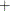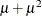### RESTRICT Statement

RESTRICT restriction1 <, restriction2 …> ;

The RESTRICT statement imposes linear restrictions on the parameter estimates. You can specify any number of RESTRICT statements.

Each restriction is written as an expression, followed by an equality operator (=) or an inequality operator (<, >, <=, >=), followed by a second expression:

expression operator expression

The operator can be =, <, >, <=, or >=.

Restriction expressions can be composed of parameter names, constants, and the operators times (), plus (), and minus (). The restriction expressions must be a linear function of the parameters. For continuous regressors, the names of the parameters are the same as the corresponding variables. For a regressor that is a CLASS variable, the parameter name combines the corresponding CLASS variable name with the variable level. For interaction and nested regressors, the parameter names combine the names of each regressor. The names of the parameters can be seen in the OUTEST= data set.

Lagrange multipliers are reported in the “Parameter Estimates” table for all the active linear constraints. They are identified with the names Restrict1, Restrict2, and so on. The probabilities of these Lagrange multipliers are computed using a beta distribution (LaMotte 1994). Nonactive (nonbinding) restrictions have no effect on the estimation results and are not noted in the output.

The following RESTRICT statement constrains the negative binomial dispersion parameterto 1, which restricts the conditional variance to be:

restrict _Alpha = 1;